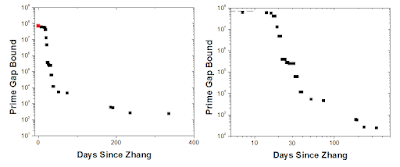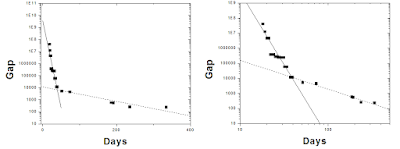## Wednesday, 1 June 2016

### The Fall of the Twin Prime Gap

The twin prime conjecture states that there are an infinite number of primes separated by a gap of two, e.g. 17 and 19 or 29 and 31. In May 2013, a relatively unknown mathematician named Yitang Zhang proved that there are infinitely primes separated by a gap of some number smaller than 70,000,000. This number is much bigger than the postulated two, but much smaller than infinity, which is where the number theory community was stuck at before. This finding heralded in a rush of work attempting to lower this bound, causing it to drop rapidly over the next several months, finally coming to a rest at 246 less than a year later, where it has sat. This post is about cataloguing and quantifying this drop.Timeline of the twin prime gap, on log-linear and log-log axes.
Most of this work was carried out by the Polymath Project, which is a group of mathematicians that collaborate on unsolved math problems. The results have been catalogued here, which is where I got the data for those graphs. The Polymath Project can be really interesting to read through, because you can see chunks of the problem getting solved in real time on an online discussion forum, like reading the world's most high-brow youtube comments. The retrospective on the project  is a nice read even for non-mathematicians such as myself. Midway through this project, an independent mathematician (working in Montreal!) named James Maynard developed another method to shrink this gap, which was eventually worked into the project.

I will not say too much about the techniques that were used to prove these bounds; there are people who are more qualified to do so who have already done so, such as Terry Tao, who was part of the project. From what I gather, the  method involving generating a large list of numbers and then "sieving" them until the solution converges*. Two assumptions can be made to make the bound easier to shrink: the  Elliott-Halberstam conjecture, and Deligne's Theorem. While their validity in this situation is unproven, assuming the  Elliott-Halberstam can reduce the bound to 6. I have tried to take only data that does not rely on these conjectures (the proven bound, if you will), and have ignored data marked as conditional in the table.

Ok, so let's look at the falling bound. There are clearly three phases: the "let's get the ball rolling" phase, the wild-mathematical-rollercoaster phase, and the "honeymoon is over" phase. The first two weeks were just people starting to realize what could be done (Zhang kind of caught the mathematical community by surprise). In fact, the first post-Zhang paper was called "A poor man's improvement on Zhang's result" and subsequently there was a blog post called "I just can’t resist: there are infinitely many pairs of primes at most 59470640 apart."  Then Terry Tao proposed the Polymath Project, and that's when it really started dropping. If you look through their website, the progress involves people posting tables of their iterated sieving data and discussing it; sometimes it works and sometimes it doesn't.

In my line of work, data is described by two types of functions: power laws and exponential curves, both of which look like straight lines if you have the right number of logarithmic axes (or the power is 1 or the x-variable covers a small range). Exponentials crop up more when something is varying with time (e.g. the length of the DNA molecule I'm looking at), while power laws come up when looking at how one quantity varies with another (e.g. how stretched a molecule is vs how confined it is). A big part of my thesis was demonstrating how we shouldn't just blindly look at power law fits when analyzing data, and that is exactly what I'm going to do now.

When I fit the steep part of the bound dropping curve to a power law, the exponent is -9.6 with respect to time. That's pretty crazy; it falls 1000 times as much in a fortnight compared to a week. If instead I fit it to exponential decay, I find that the characteristic time for the gap to fall by a factor of e is about three days, although this doesn't fit the data as well as the power law. I have also ignored the slowdown that occurred around day 30 (although, within a month of Zhang's news, they had already dropped by bound by a factor of 300).

The results sort of petered out in the thousands, and then they were re-invigorated by Maynard's discoveries, which is what got them down to 246. If I do the same type of analysis for the slow phase, there is an inverse-square decay of the gap with respect to time, or a characteristic exponential decay of about 72 days. Can this teach us anything about the nature of collaborative number theory? Probably not.The gap data with unjustified exponential (left) and power law (right) fits to the fast and slow portions of the drop.

I was watching this gap fall as it was happening, and I was curious as to why it stopped at the seemingly arbitrary 246. A googling came up with an excellent reddit comment explaining it from a user named BoundingBadger. It was pretty neat seeing an unsolved problem get hammered down by professional and sometimes famous mathematicians in real time and openly discussing their results, and I'm glad I caught that neat little bit of history. I was curious as to how the bound would end up behaving over time, and now I know!

*That is a really bad explanation but it's the limit of my understanding right now.